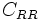# Rolling resistance

The magnitude of rolling resistance depends on the nature of the surfaces in contact and the magnitude of the normal force. The constant of proportionality$C_{RR}$ such that the rolling resistance is$C_{RR}$ times the normal force is termed the rolling resistance coefficient, and its magnitude is generally less than$0.01$.